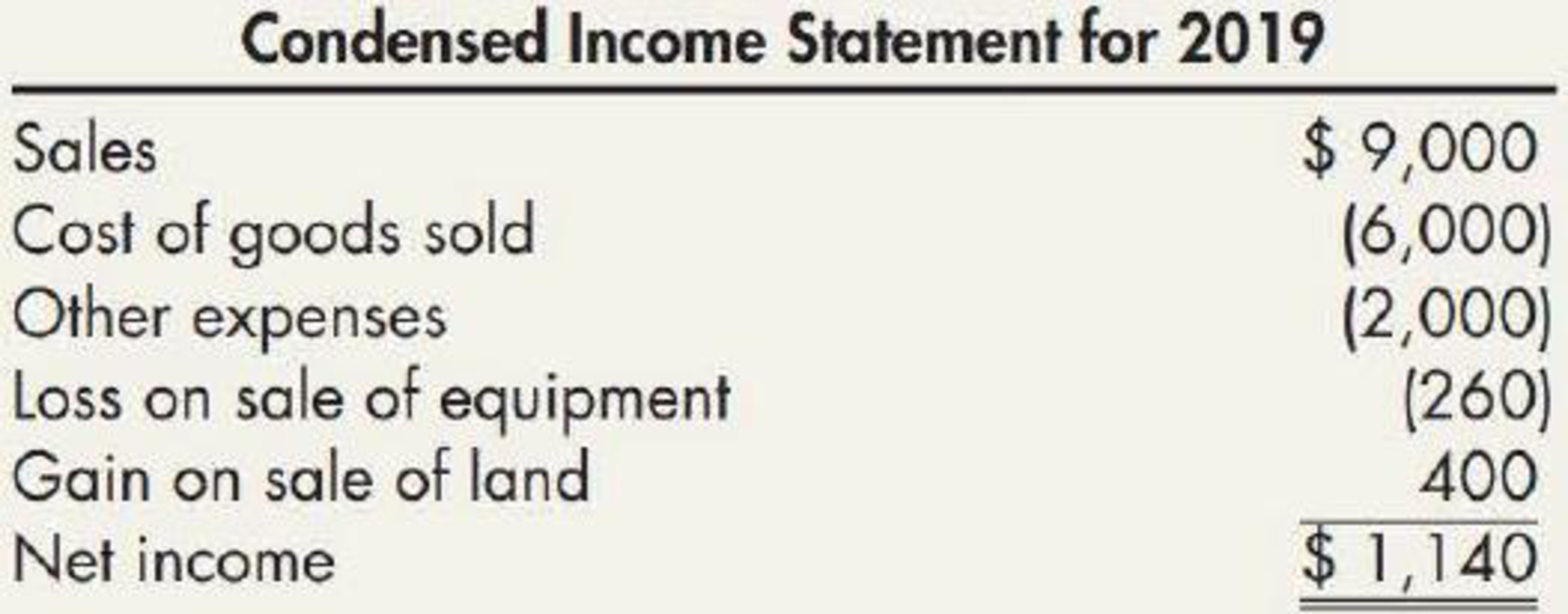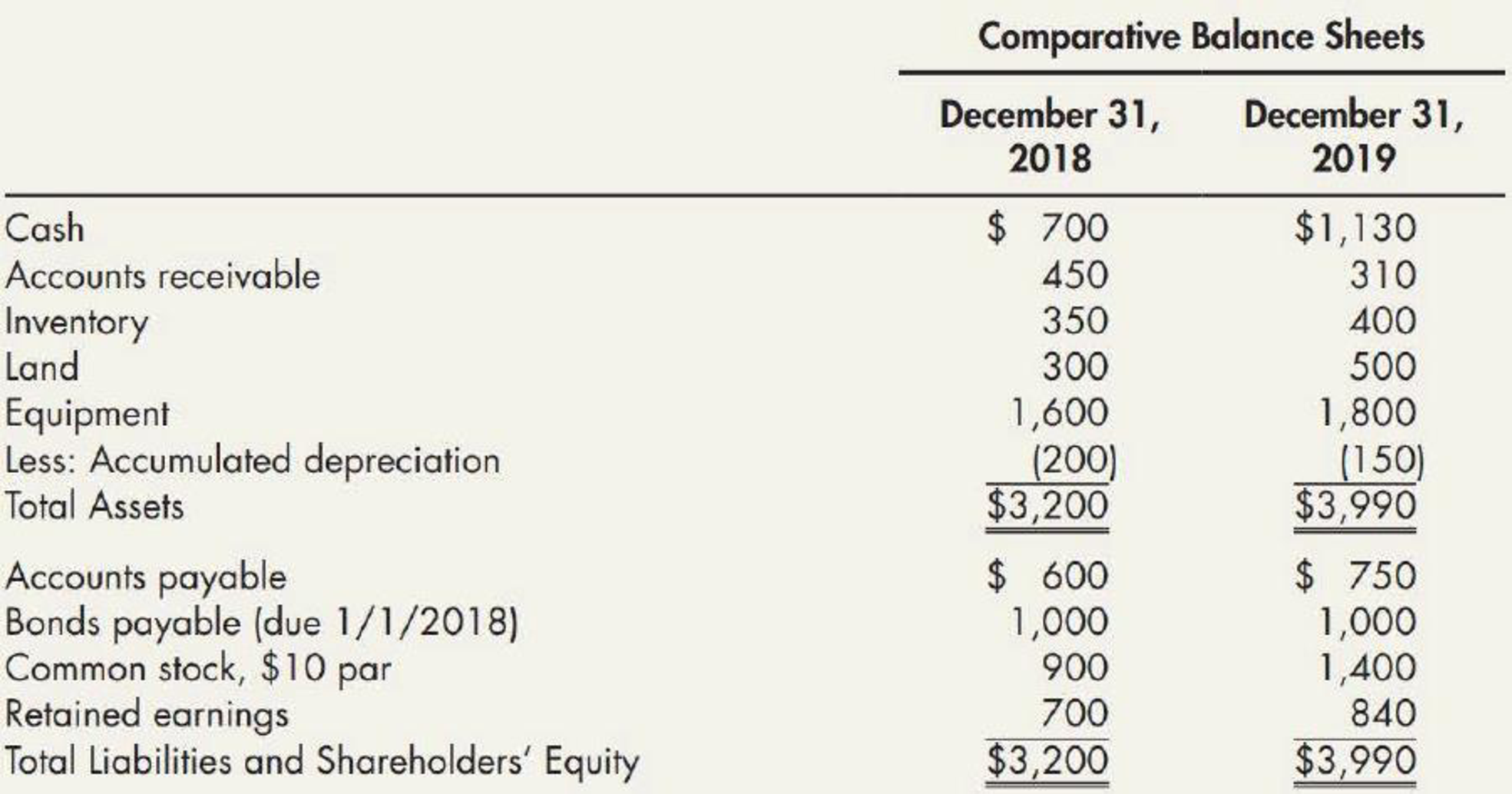Chapter 21, Problem 11E### Intermediate Accounting: Reporting...

3rd Edition
James M. Wahlen + 2 others
ISBN: 9781337788281

#### Solutions

Chapter
Section### Intermediate Accounting: Reporting...

3rd Edition
James M. Wahlen + 2 others
ISBN: 9781337788281
Textbook Problem
18 views

# Spreadsheet and Statement The following 2019 information is available for Stewart Company:Partial additional information: The equipment that was sold for cash had cost $400 and had a book value of$300. Land that was sold brought a cash price of $530. Fifty shares of stock were issued at par. Required:Making whatever additional assumptions that are necessary, 1. Prepare a spreadsheet to support a 2019 statement of cash flows for Stewart. 2. Prepare the statement of cash flows. 1. To determine Prepare the cash flow statement under spread sheet method of S company for the year ended 2019. Explanation Statement of cash flows: Cash flow statement reports all the cash transactions which are responsible for inflow and outflow of cash, and result of these transactions is reported as ending balance of cash at the end of reported period. Statement of cash flows includes the changes in cash balance due to operating, investing, and financing activities. Worksheet: A worksheet is a spreadsheet used while preparing a financial statement. It is a type of form having multiple columns and it is used in the adjustment process. The use of a worksheet is optional for any organization. A worksheet can neither be considered as a journal nor a part of the general ledger. Prepare the cash flow statement under spread sheet method. Table (1) Working Note: 1. (a) Net income for the year ended 2019 is$1,140.
2. (b) Calculate the decrease in accounts receivable.

Decrease in accounts receivable = (opening accounts receivable – closing accounts receivable) = $450-$310=$140 1. (c) Calculate an increase in inventory. Increase in inventory = Closing inventory - opening inventory=$450-$310=$50

2. (d) Calculate the cost of land sold.

Cost of land sold = (Proceeds from sale of land - Gain on sale of land)=$530$400=$130 3. (e) Calculate the purchase of land. Purchase of land = (Closing balanceBalance amount of land after sale)=$500$170=$330

Balance amount of land after sales)={Opening balanceSale of land}=$300$130=$170 1. (f) Calculate the Proceeds from Equipment. Proceeds from sale of equipment = Book value Selling cost=$300$260=$40

2. (g) Calculate the Purchase of equipment

2.

To determine

Prepare the cash flow statement of S company for the year ended 2019.

### Still sussing out bartleby?

Check out a sample textbook solution.

See a sample solution

#### The Solution to Your Study Problems

Bartleby provides explanations to thousands of textbook problems written by our experts, many with advanced degrees!

Get Started

#### Find more solutions based on key concepts